﻿ 地震和波浪共同作用下斜坡式防波堤的动力响应分析
«上一篇文章快速检索 高级检索

 应用科技2019, Vol. 46Issue (2): 19-24  DOI: 10.11991/yykj.2018070090

引用本文ZHANG Yunce, ZHANG Guixin, MAO Jize, et al. Dynamic response analysis for mound breakwaters under the action of earthquake and wave loads[J]. Applied Science and Technology, 2019, 46(2), 19-24. DOI: 10.11991/yykj.201807009.文章历史

1. 哈尔滨工程大学 航天与建筑工程学院，黑龙江 哈尔滨 150001;
2. 中国地震局工程力学研究所，黑龙江 哈尔滨 150080

Dynamic response analysis for mound breakwaters under the action of earthquake and wave loads
ZHANG Yunce1, ZHANG Guixin2, MAO Jize1, GUO Qingyong1, LIU Zongmin11. College of Aerospace and Civil Engineering, Harbin Engineering University, Harbin 150001, China;
2. Institute of Engineering Mechanics, China Earthquake Administration, Harbin 150080, China
Abstract: For mound breakwaters, the structural analysis of dynamic response is the main method to study the seismic performance of structures. Since the mound breakwater is affected by waves during the earthquake, the seismic response analysis of the mound breakwater needs to consider the interaction of earthquake and waves. In this paper, the corresponding finite element model is established by using ABAQUS software, and the model is verified by comparison. The dynamic response of the mound breakwater under the combined action of earthquake and the wave is simulated. The results show that the interaction between earthquake and the wave has a significant effect on the vertical residual deformation of the mound breakwater structure compared with the individual earthquake action. Waves will have different effects on the vertical residual deformation of the mound breakwater structure.
Keywords: earthquake action    wave action    mound breakwater    dynamic response    finite element analysis    numerical simulation    constitutive model    Rayleigh damping

1 基本方程

 $\begin{array}{l} { M}{{\ddot u}_s}\left( t \right) + { C}{{\dot u}_s}\left( t \right) + { K}{u_s}\left( t \right) = - { M}{{\ddot u}_g}\left( t \right) + p + P \end{array}$

 $\begin{array}{l} {p_{x = 0}}\left( {y,t} \right) = \displaystyle\frac{{4{{\ddot u}_0}\rho }}{{\text π} }\cos \omega t\sum\limits_{n = 1,3,5, \cdots }^\infty {\frac{1}{{\sqrt {\lambda _n^2 - \displaystyle\frac{{{\omega ^2}}}{{{c^2}}}} }}\sin {\lambda _n}y} \end{array}$

 \left\{ {\begin{aligned} & {{P_{{h_1}}} = \alpha \rho g{h_1}}\\ & {{h_1} = {h_w}\left( {1 - \frac{Z}{{{R_u}}}} \right)}\\ & {\alpha = 2.9{{\left( {\frac{{{R_u}}}{{{H_u}}}\cos \;\; \beta } \right)}^2}} \end{aligned}} \right.

 ${u_q} - {u_s} = 0 \;\; \left( {{\text{在}}{S_{qs}}{\text{上}}} \right)$

 ${T_q} + {T_s} = 0 \;\; \left( {{\text{在}}{S_{qs}}{\text{上}}} \right)$

2 斜坡式防波堤的有限元模型 2.1 参数及边界的选择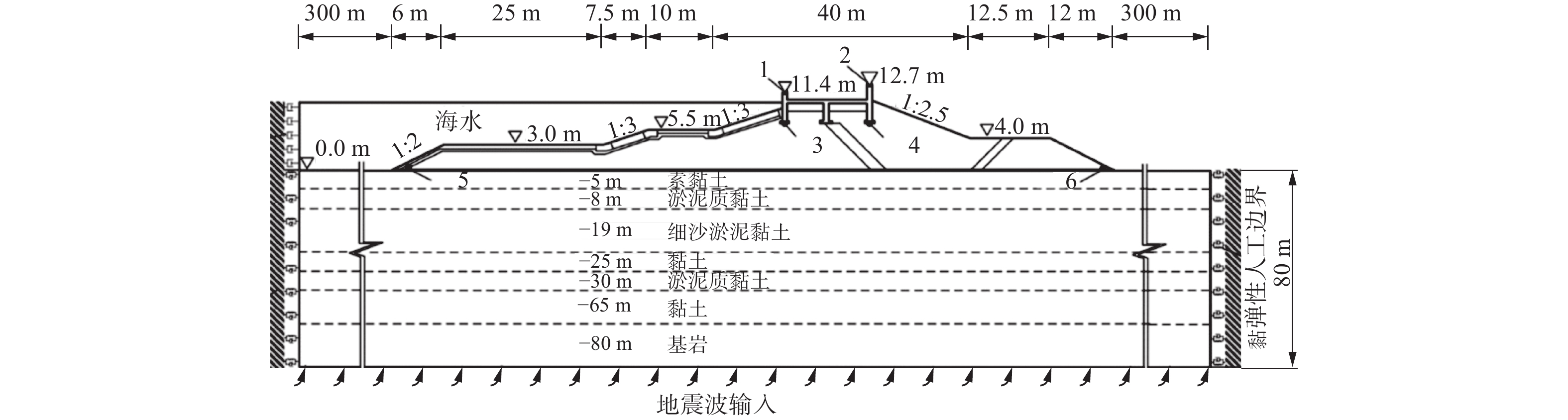Download: 图 1 斜坡式防波堤结构和地基土层的整体断面示意表 1 土层参数
2.2 有限元建模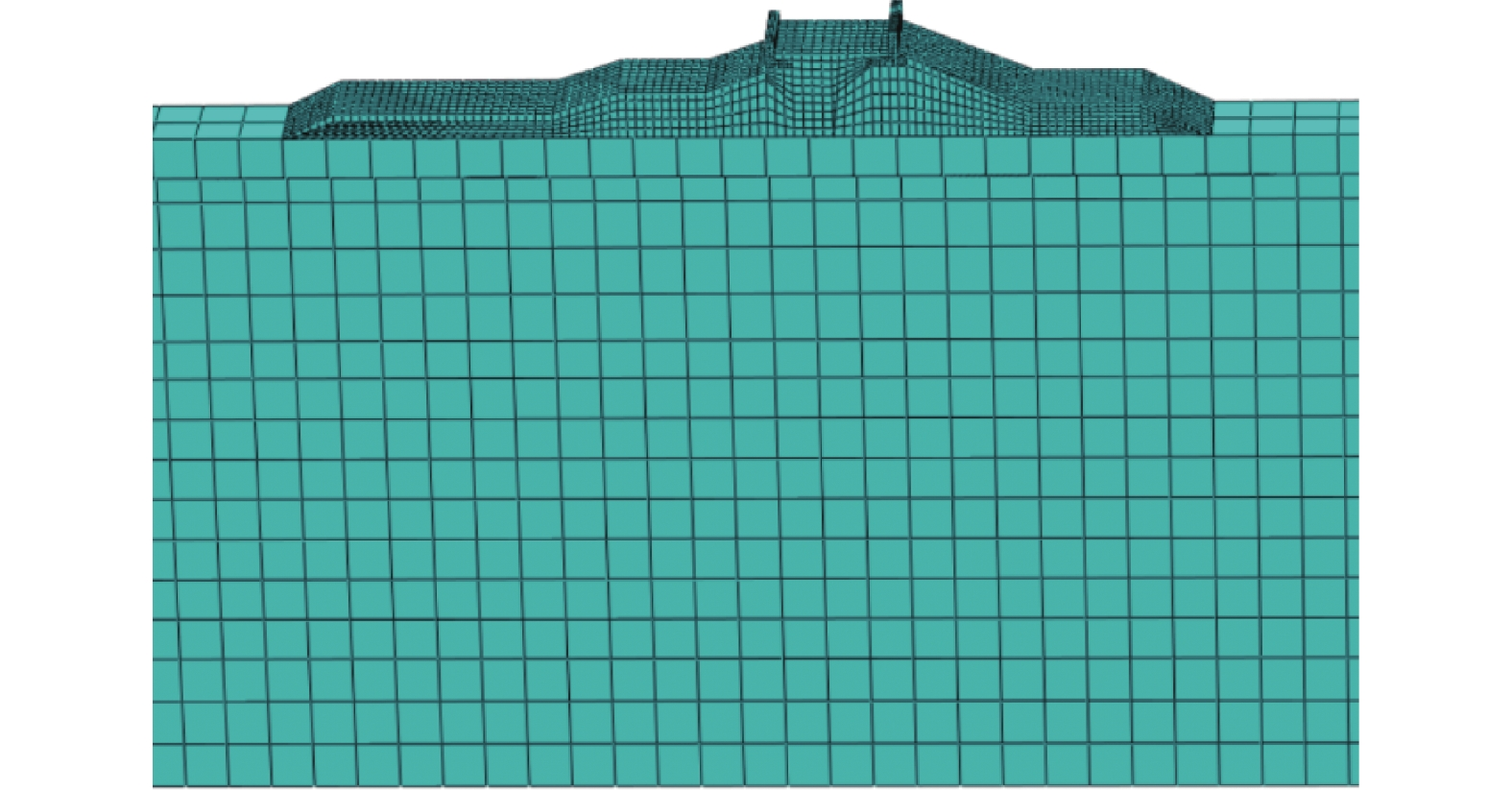Download: 图 2 模型网格划分
2.3 本构模型及阻尼的选择

 ${ C} = \alpha { M} + \beta { K}$

 $\alpha = \frac{{2{\omega _{\text{i}}}{\omega _j}({\xi _i}{\omega _j} - {\xi _j}{\omega _i})}}{{\omega _j^2 - \omega _i^2}}$
 $\beta = \frac{{2({\xi _j}{\omega _j} - {\xi _i}{\omega _i})}}{{\omega _j^2 - \omega _i^2}}$

3 斜坡式防波堤的动力响应分析 3.1 荷载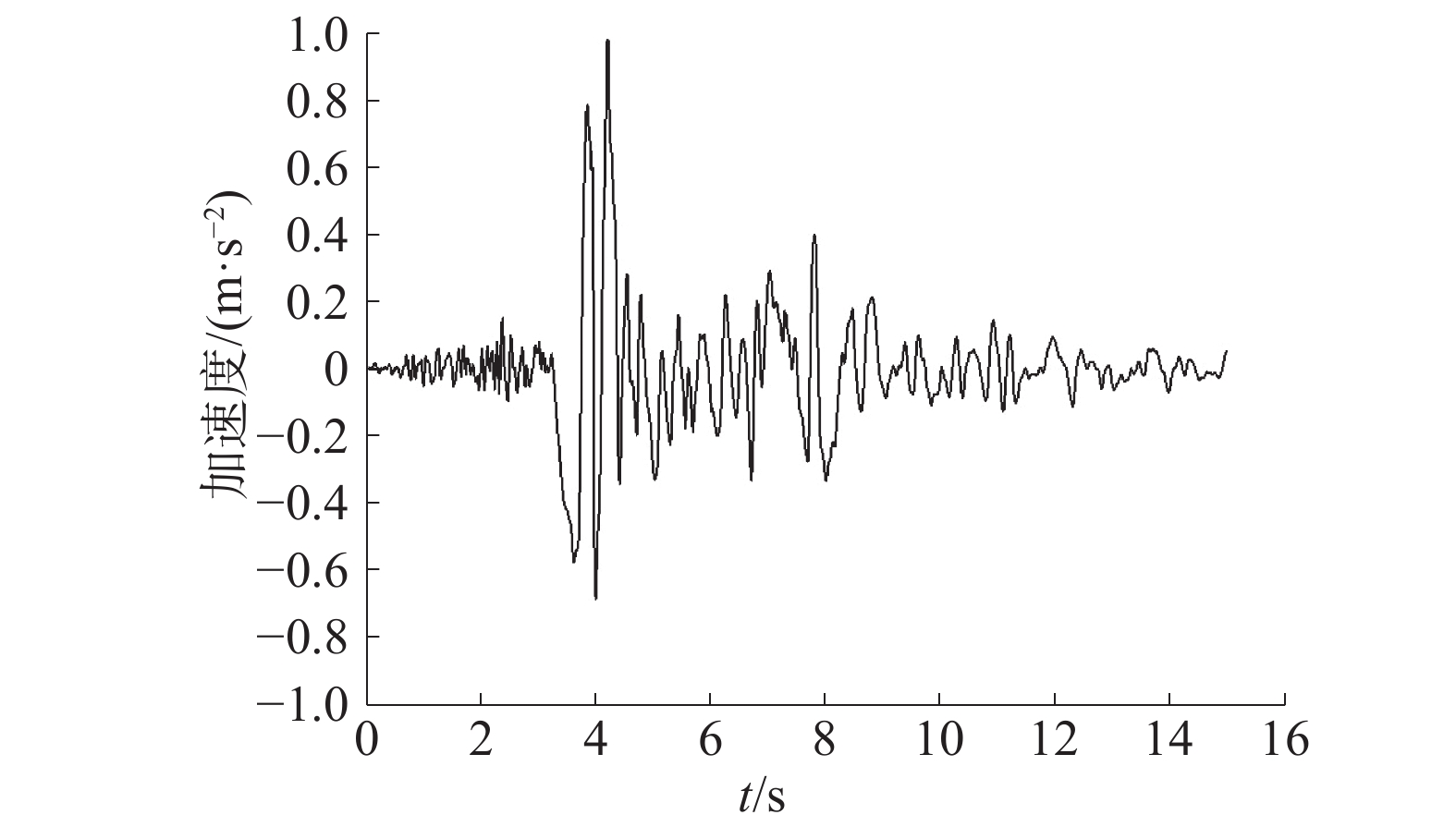Download: 图 3 Frulli地震波加速度时程曲线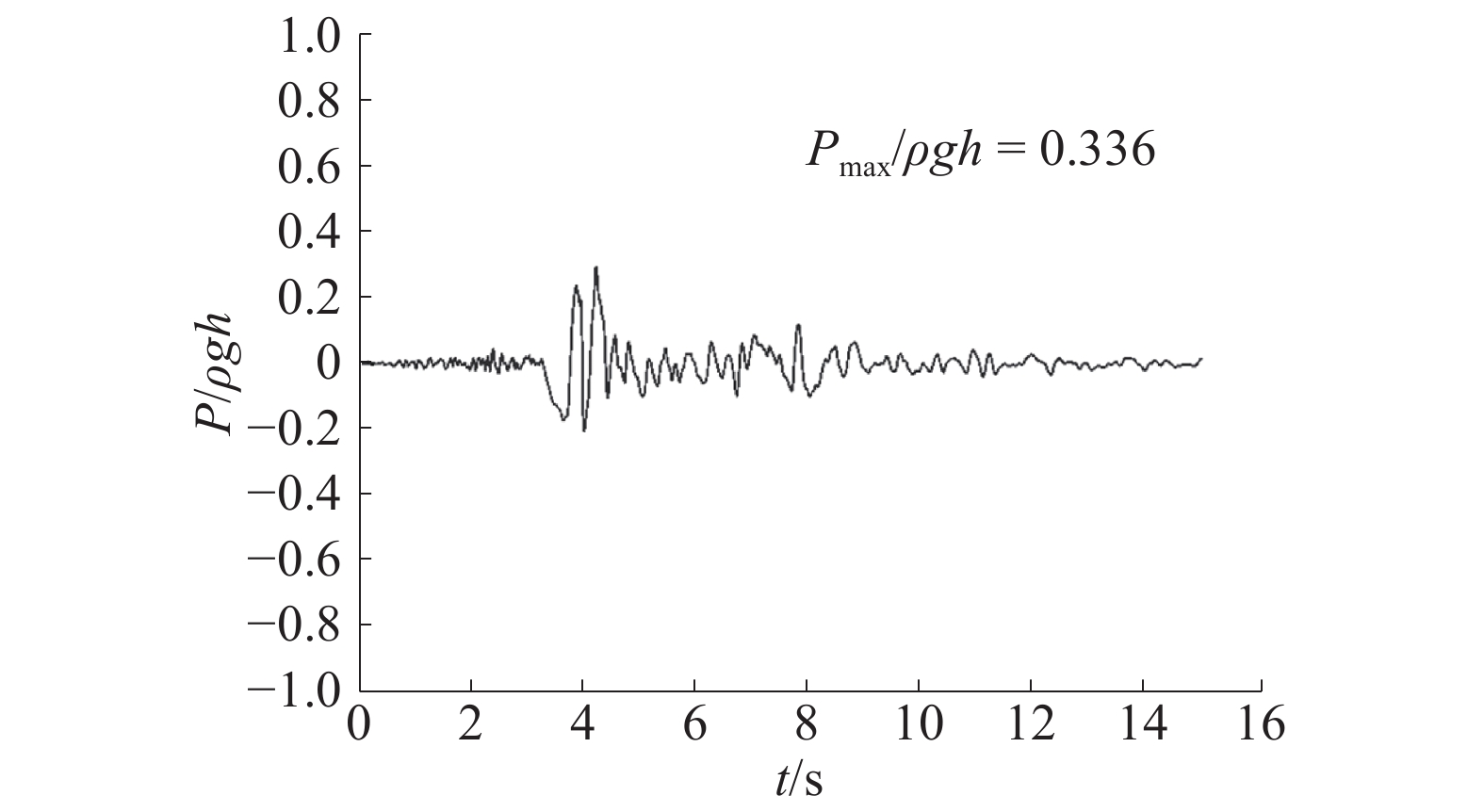Download: 图 4 $y = h$ 时，动水压力时程曲线
3.2 模型的验证和对比表 2 斜坡式防波堤的残余变形与文献比较

3.3 地震和波浪共同作用下斜坡式防波堤的动力响应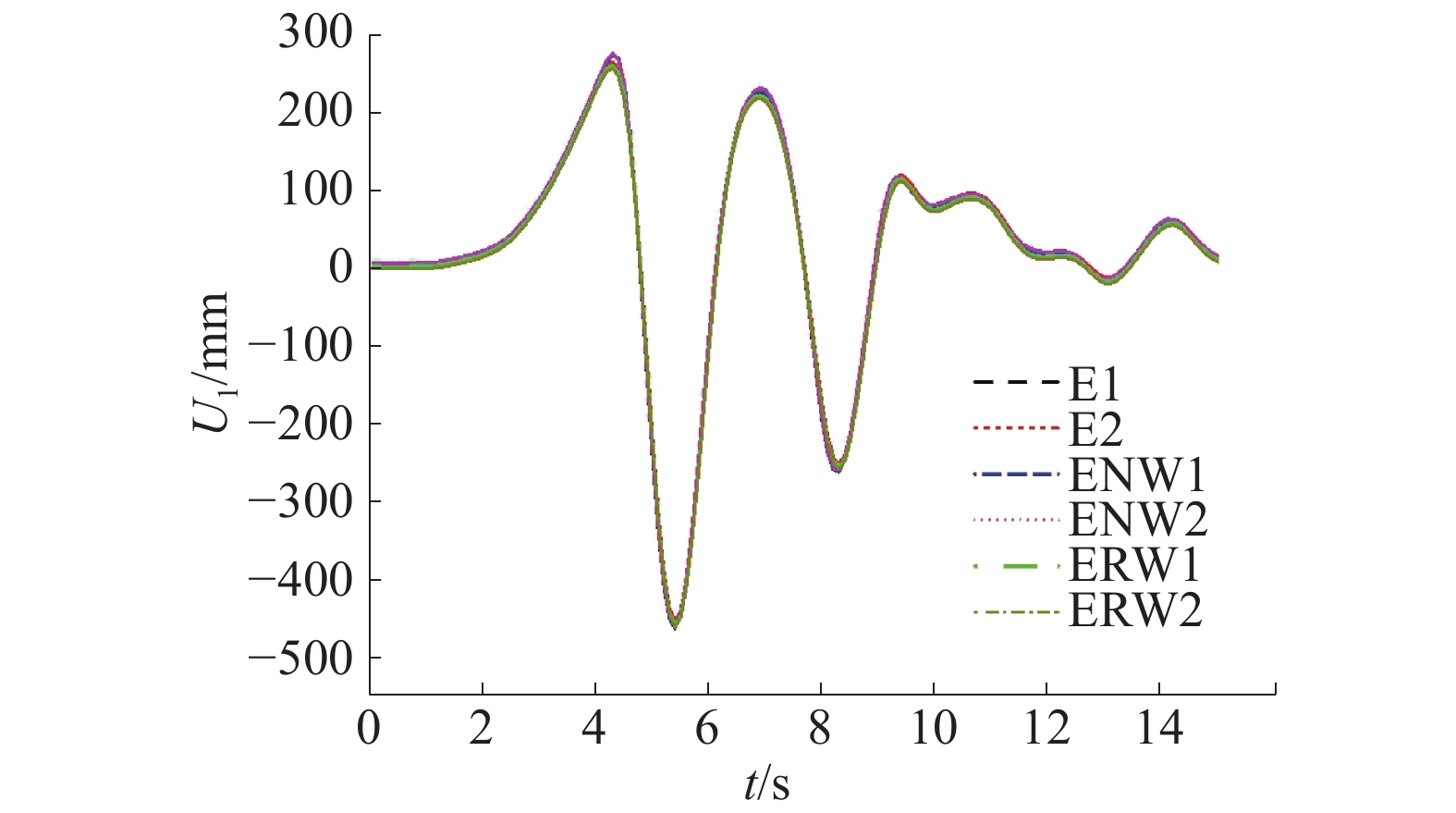Download: 图 5 x方向1点和2点位移曲线Download: 图 6 x方向5点和6点位移曲线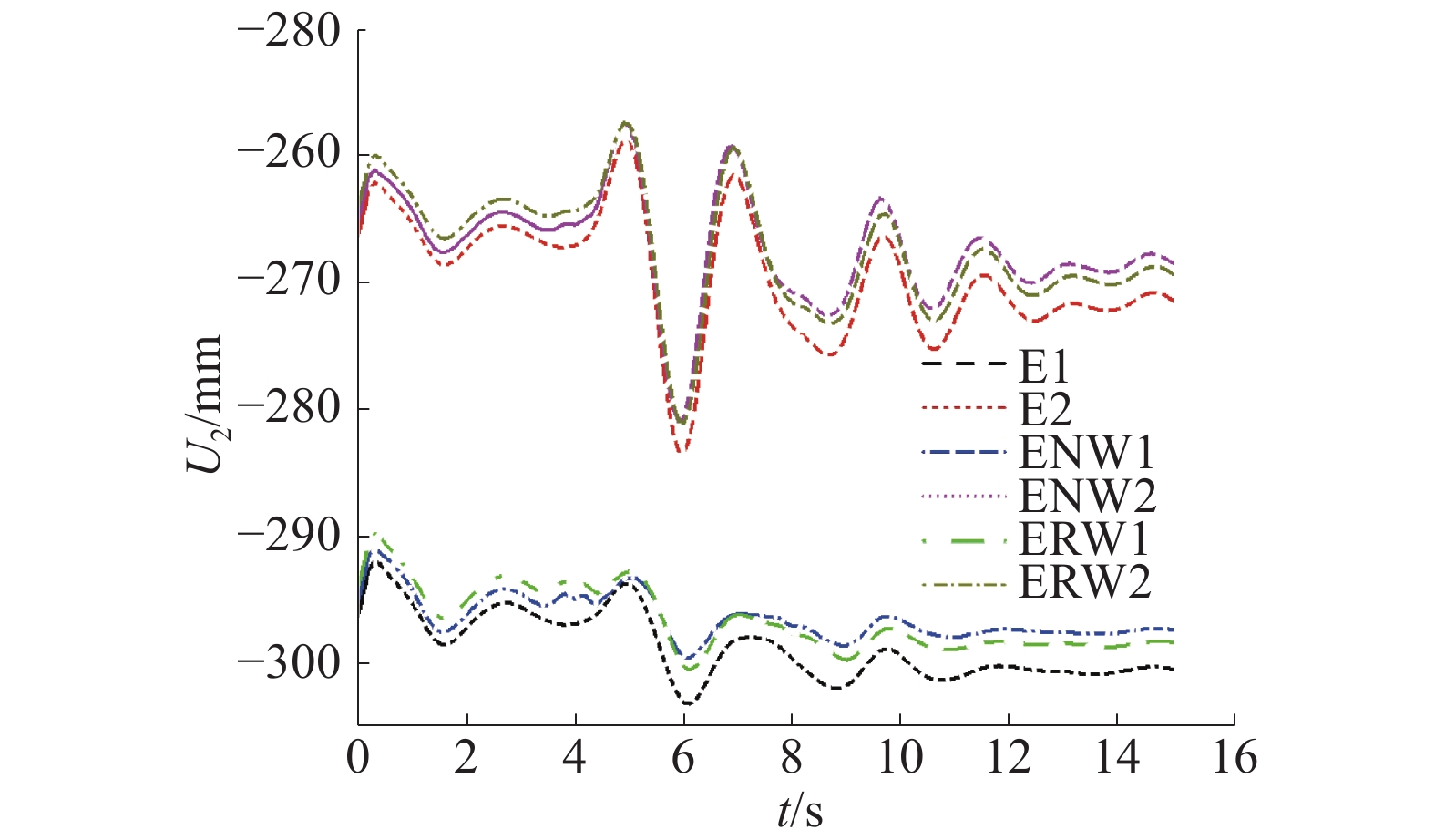Download: 图 7 y方向1点和2点位移曲线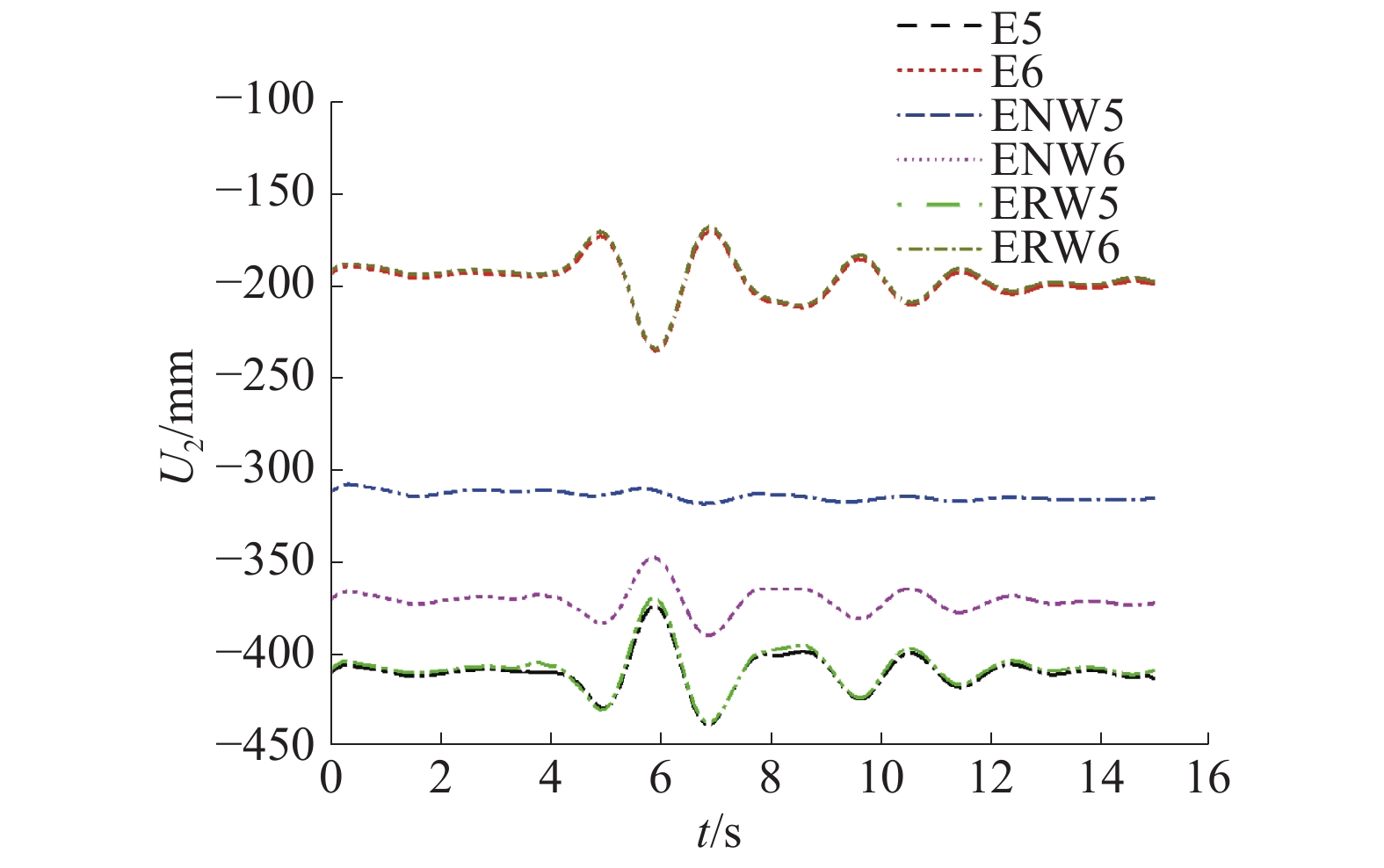Download: 图 8 y方向5点和6点位移曲线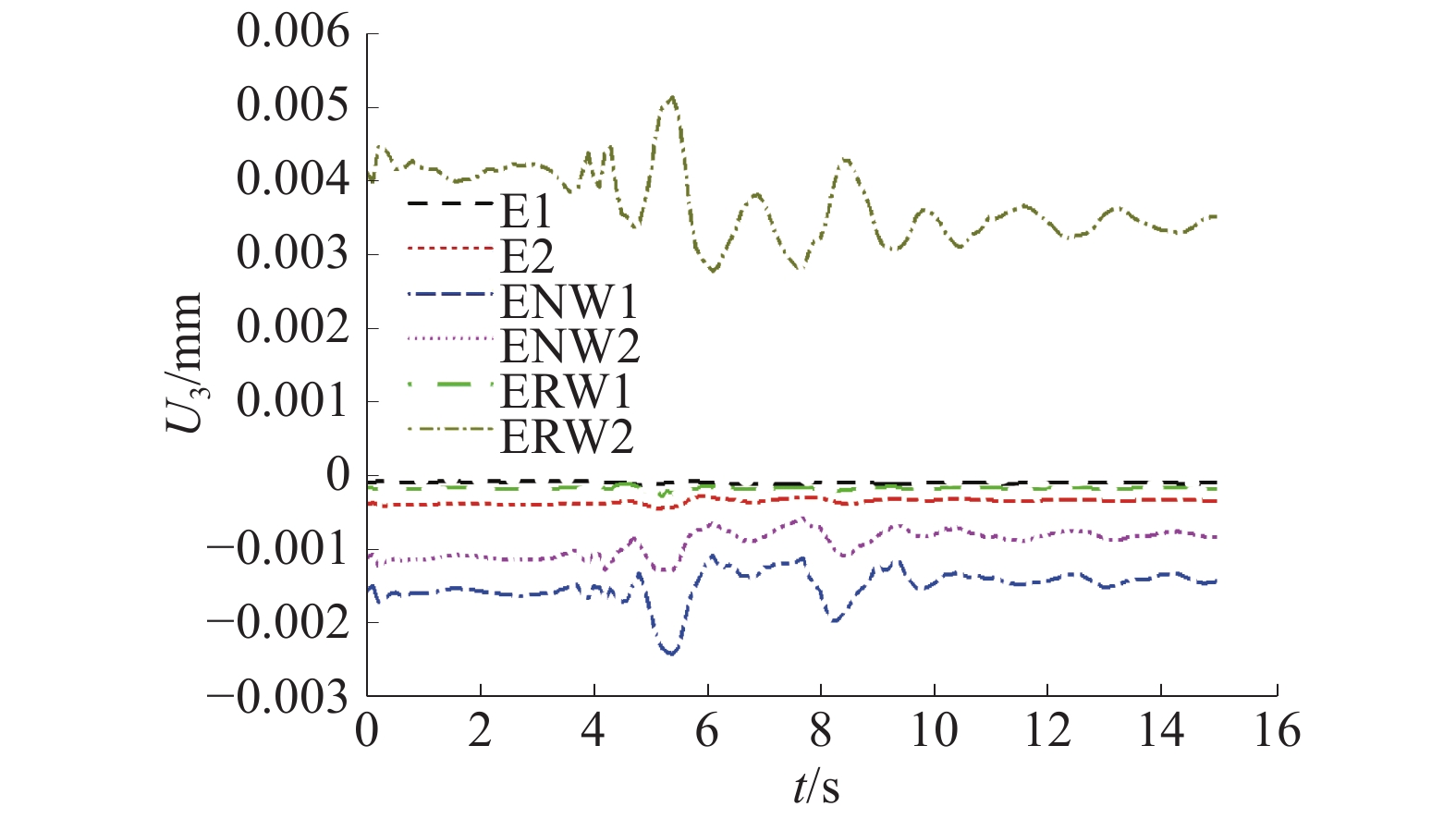Download: 图 9 z方向1点和2点位移曲线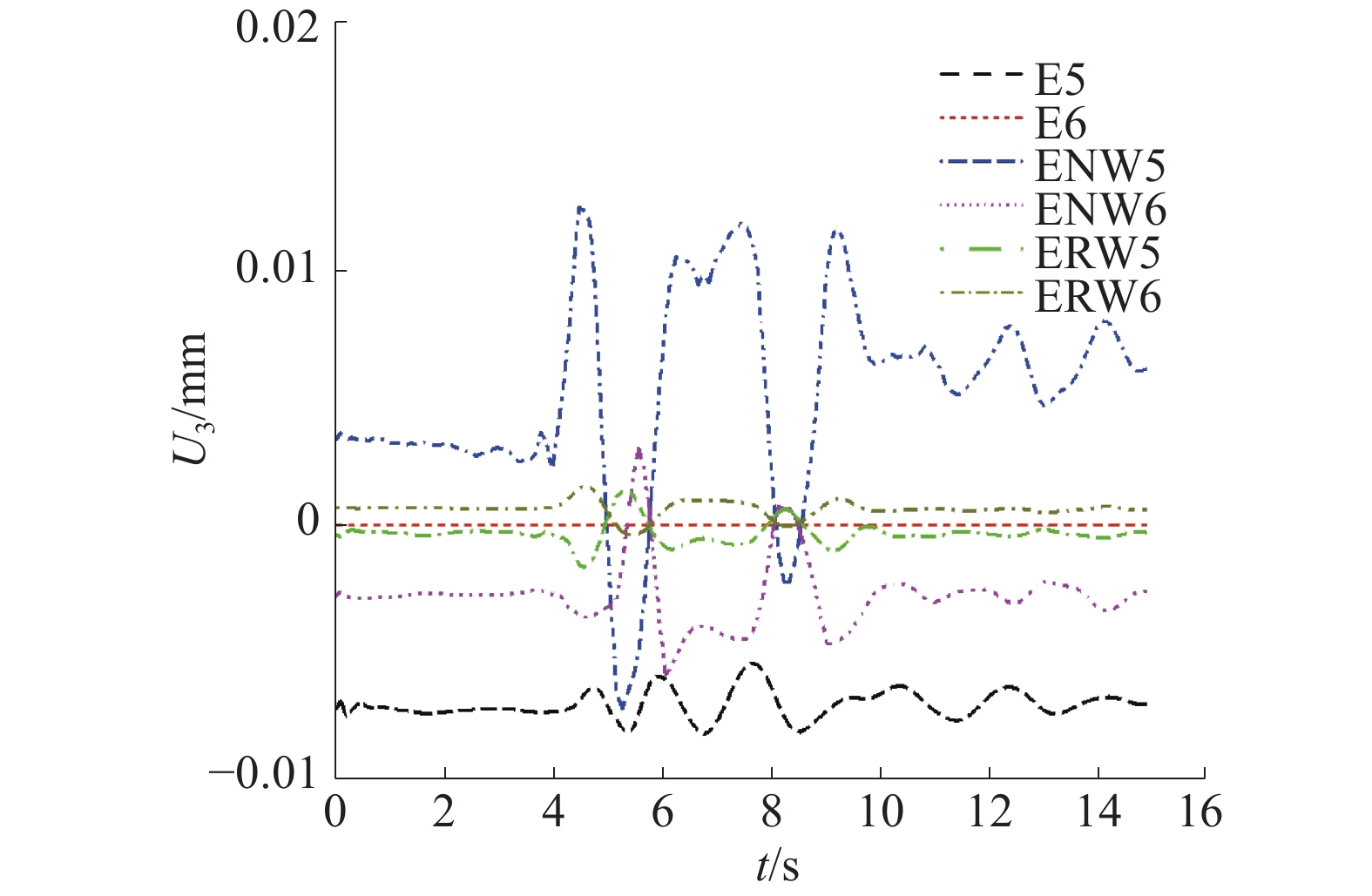Download: 图 10 z方向5点和6点位移曲线

3.4 地震和波浪共同作用下斜坡式防波堤的竖向残余变形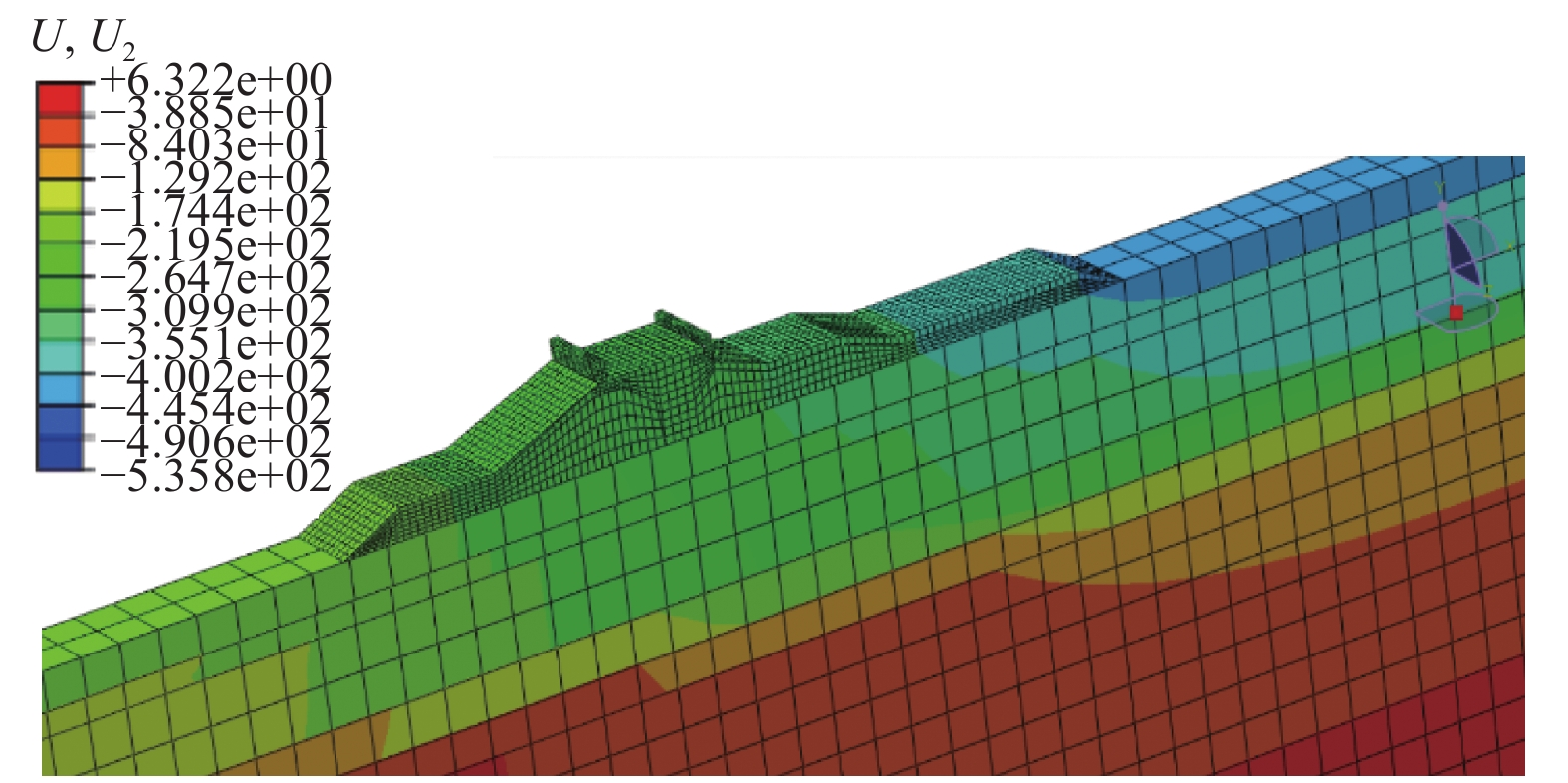Download: 图 11 地震作用下竖向残余变形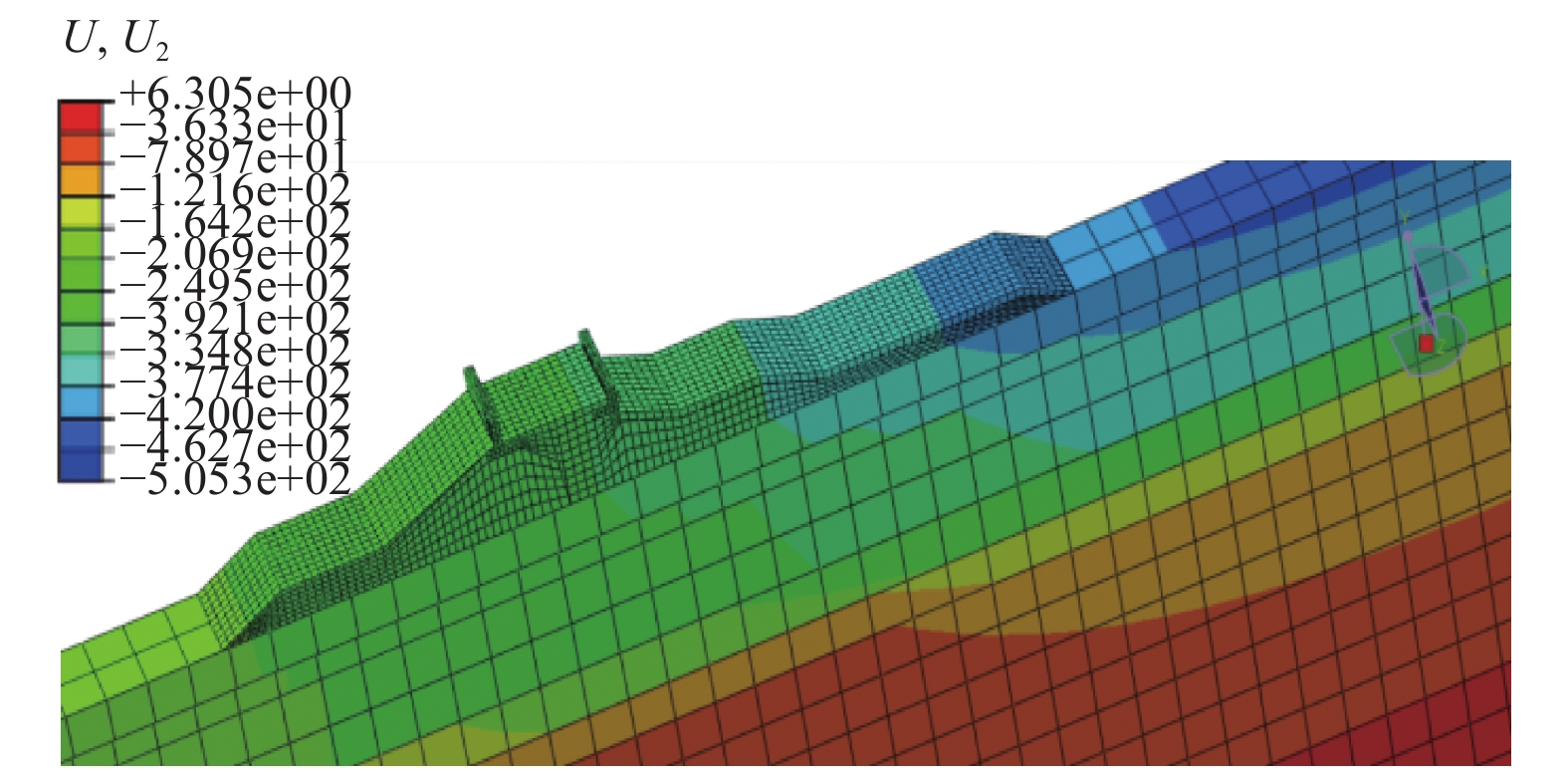Download: 图 12 地震和总波浪力共同作用下竖向残余变形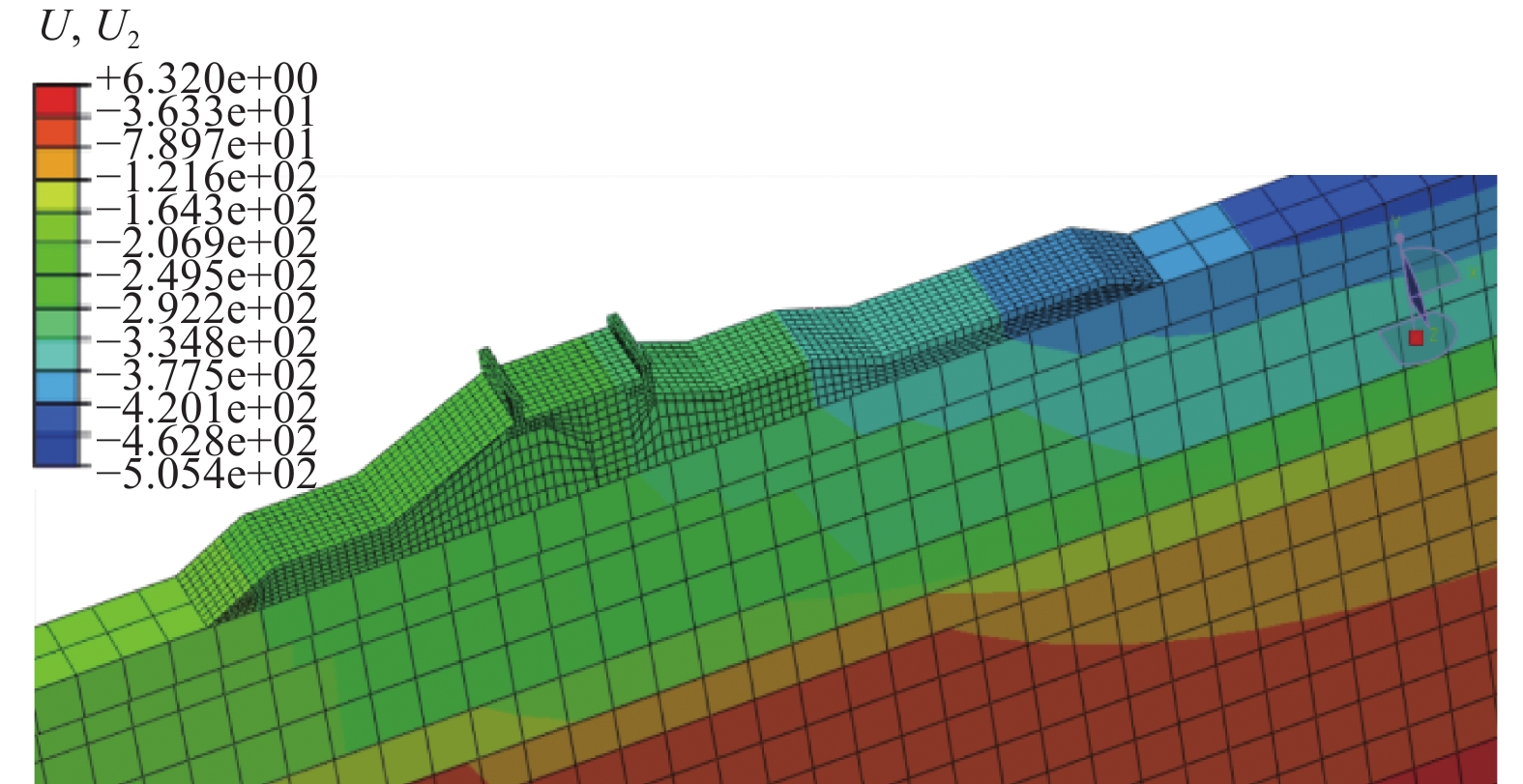Download: 图 13 地震和规则波共同作用下竖向残余变形表 3 不同荷载作用下的竖向残余变形

4 结论

1）在地震和波浪共同作用下，斜坡式防波堤残余变形机理十分复杂。向海侧坡脚在地震和波浪共同作用下的竖向残余变形大于单独地震作用下的竖向残余变形；而前后挡浪墙和背海侧坡脚在地震和波浪共同作用下的竖向残余变形均小于单独地震作用下的竖向残余变形。由此可见，地震发生时波浪的存在将对斜坡式防波堤结构竖向残余变形造成不同程度的影响。

2）波浪荷载分别按总波浪力和规则波的方式加载，但从竖向残余变形的仿真结果来看，两者的差别很小。

3）地震和波浪共同作用与单独地震作用相比，斜坡式防波堤结构的水平残余变形无明显差异，所以水平方向的残余变形只考虑地震荷载即可。

  杨勋, 王欢欢, 余克勤, 等. 流−固耦合作用下斜坡式防波堤地震动力响应分析[J]. 工程力学, 2016, 33(10): 248-256. (0)  YE J H, JENG D S. Three-dimensional dynamic transient response of a poro-elastic unsaturated seabed and a rubble mound breakwater due to seismic loading[J]. Soil dynamics and earthquake engineering, 2013, 44: 14-26. DOI:10.1016/j.soildyn.2012.08.011 (0)  YANG Xun, JIN Xianlong. Dynamic analysis of a rubble-mound breakwater subject to earthquake including seawater-structure-foundation interaction[J]. Proceedings of the institution of mechanical engineers, part M: journal of engineering for the maritime environment, 2015, 230(2): 310-326. (0)  OKADA K, SUZUKI K, ARIKAWA T. Study of a force to act on a breakwater at the superposition of an earthquake and the tsunami[J]. Journal of Japan society of civil engineers Ser B2 (coastal engineering), 2016, 72(2): I_1057-I_1062. DOI:10.2208/kaigan.72.I_1057 (0)  武崇福, 刘贝贝. 波浪和地震作用下高桩承台−土−结构动力响应[J]. 土木建筑与环境工程, 2011, 33(6): 7-12, 58. (0)  何晓宇, 李宏男. 波浪与地震对小尺度桩柱的共同作用研究[J]. 地震工程与工程振动, 2007, 27(5): 139-145. DOI:10.3969/j.issn.1000-1301.2007.05.021 (0)  李忠献, 黄信. 地震和波浪联合作用下深水桥梁的动力响应[J]. 土木工程学报, 2012, 45(11): 134-140. (0)  柳国环, 练继建, 于通顺. 地震与波浪作用下近海风电结构响应及倒塌模式[J]. 应用基础与工程科学学报, 2016, 24(1): 71-80. (0)  荣维栋, 李洪斌. 近海单桩风机在波浪地震联合作用下的动力特性分析[J]. 江苏科技大学学报(自然科学版), 2015, 29(1): 27-32. DOI:10.3969/j.issn.1673-4807.2015.01.005 (0)  何晓宇, 李宏男. 地震与波浪联合作用下海洋平台动力特性分析[J]. 海洋工程, 2007, 25(3): 18-25. DOI:10.3969/j.issn.1005-9962.2007.03.006 (0)  CHAUDHARY B, HAZARIKA H, ISHIBASHI I, et al. Sliding and overturning stability of breakwater under combined effect of earthquake and tsunami[J]. Ocean engineering, 2017, 136: 106-116. DOI:10.1016/j.oceaneng.2017.03.021 (0)  柳春光, 张士博. 考虑地震、波浪和海流作用的跨海桥梁结构研究进展[J]. 大连理工大学学报, 2017, 57(1): 105-110. (0)  居荣初, 曾心传. 弹性结构与液体的耦联振动理论[M]. 北京: 地震出版社, 1983. (0)  沈靠山, 李翔. 秦山核电厂海堤加高工程挡浪墙波浪力研究[J]. 浙江水利科技, 2013, 41(3): 53-54. DOI:10.3969/j.issn.1008-701X.2013.03.019 (0)  王艳. 基于ABAQUS的混凝土重力坝地震响应仿真分析[D]. 杨凌: 西北农林科技大学, 2010. (0)  中国水电水利科学研究院. DL 5073-2000, 水工建筑物抗震设计规范[S]. 北京: 中国电力出版社, 2001. (0)